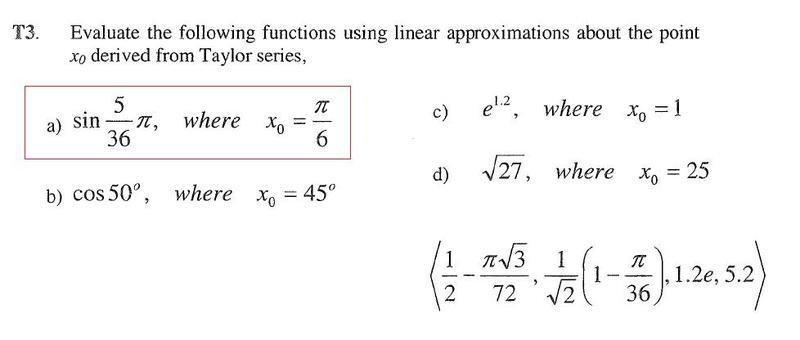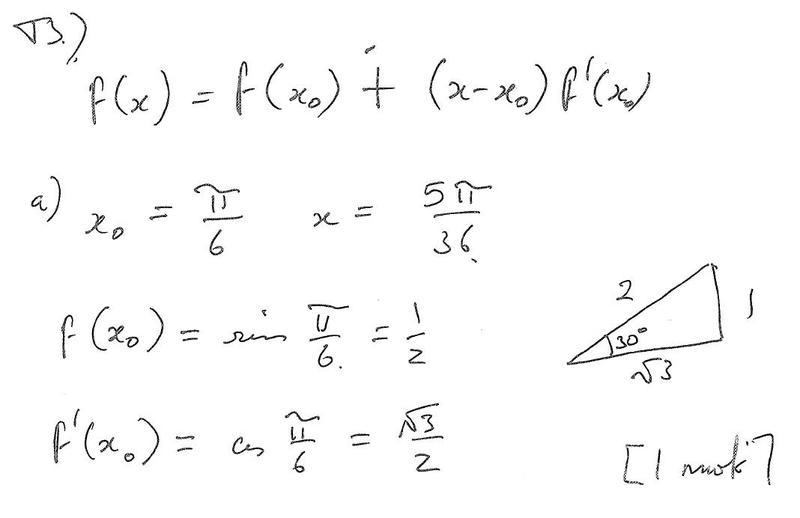# Linear approximations derived from Taylor series

## Homework Statement

So I have the problem questiona dn my teachers solution posted below.
I understand:

f(xo) = sin pi/6
f '(xo) = cos pi/6

but i dont know how he gets them into fraction form with the SQRT of 3, it looks like some pythagoras but i dont really know how he did it.

## Homework Equations## The Attempt at a Solutiontiny-tim
Homework Helper
Hi _Greg_!(have a pi: π)
f(xo) = sin pi/6
f '(xo) = cos pi/6

but i dont know how he gets them into fraction form with the SQRT of 3, it looks like some pythagoras but i dont really know how he did it.

I can't see the pictures yet, but π/6 = 30º, which is the little angle of half an equilateral triangle, so sin(π/6) (opp/hyp) = 1/2, and yes from Pythagoras you get cos(π/6) = √3/2.Thanks for the fast reply tiny tim.
But how would you know its a 30* triangle, and how do you know cos pi/6 = sqrt3/2
Like sin pi/6, is pi close enough to 3 to just call it 3/6?
I don't really know much of the basics, I'm pretty much learning this for the deep end, looking for trends to understand

tiny-tim
Homework Helper
(what happened to that π i gave you?)
But how would you know its a 30* triangle

Because π = 180º, so π/6 = 30º

(and an equilateral triangle has all angles equal, and they add up to 180º, so each angle is 60º, and half that is 30º)
Like sin pi/6, is pi close enough to 3 to just call it 3/6?

No, just draw half an equilateral triangle …

one side will be half the length of the other.

aaaaaaah, I see, I thought that little n symbol was pi.
And of course, it all adds up to 180*, high school maths is all coming back nowMany thanks